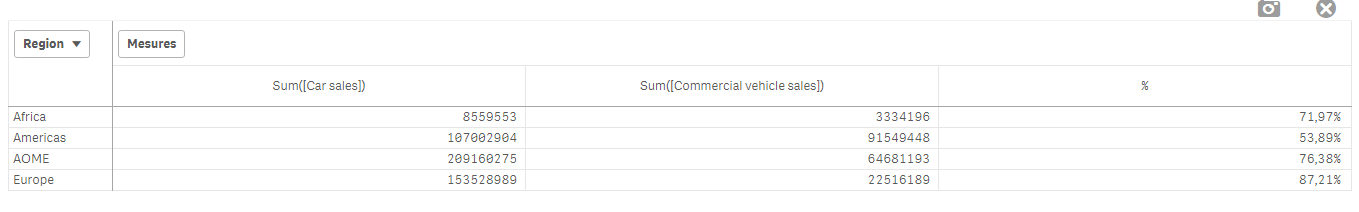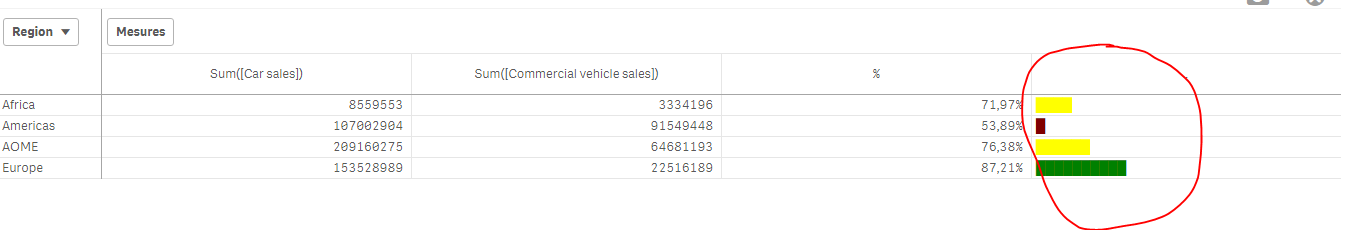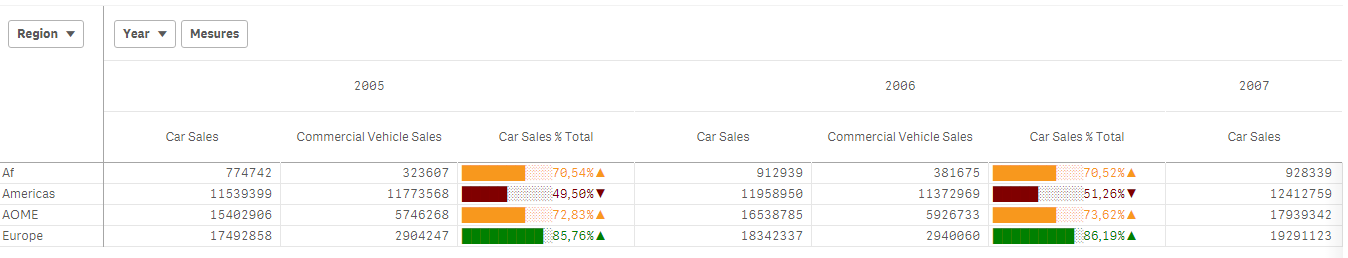# Qlik Sense Documents & Videos

Documents & videos about Qlik Sense.

Announcements
QlikWorld, June 24-25, 2020. Free virtual event for DI and DA gurus. Register Now

# Creating Mini Chart in Qlik Sense tablesPartner

## Creating Mini Chart in Qlik Sense tables

Hi all,

Mini Charts have always been a good feature in QlikView and It's really 'frustrating' that this has not been added (yet) in Qlik Sense.

With hopes that the Qlik Team will think about such improvements for the next releases, let's discuss here how to create our own mini chart in a Qlik Sense Table.

The following expressions might seem 'complicated' at first sight, but remember that It will be generic.

Thus, their use will be very easy.

Let's begin :

Suppose we have:

As a dimension: OICA Region

As measures:

Sum(Sales)

Sum("Commercial Vehicle Sales")

Sum(Sales) / (Sum(Sales)+Sum("Commercial Vehicle Sales"))

Here is our current table:Now, we want to add, a mini 'bar' to represent the %:

How to do so:

As an expression:

=repeat('█',

rangemax(rangemin( ceil(((column(1)/column(2))-1)*2.5),10),0)

)

With that, we repeat the square sign and precise the limits.

And as a text Color expression:

if( (sum([Car sales])/(sum([Car sales])+sum([Commercial vehicle sales])))>=0.8,Green(),

if((sum([Car sales])/(sum([Car sales])+sum([Commercial vehicle sales])))>=0.7,Yellow(),

Red()))

Result:We can also Add the % in front of the bar by adding it in the expression:

From :

=repeat('█',

rangemax(rangemin( ceil(((column(1)/column(2))-1)*2.5),10),0)

)

To:

=repeat('█',

rangemax(rangemin( ceil(((column(1)/column(2))-1)*2.5),10),0)

) & num((sum([Car sales])/(sum([Car sales])+sum([Commercial vehicle sales]))),'# ##0,00%')

Result:You can even alter it as follow:

repeat(chr(09608),round( sum([Car sales])/(sum([Car sales])+sum([Commercial vehicle sales])) * 10))

&repeat(chr(09617),10-round( sum([Car sales])/(sum([Car sales])+sum([Commercial vehicle sales])) *10))

&num((sum([Car sales])/(sum([Car sales])+sum([Commercial vehicle sales]))),'# ##0,00%')

&if(sum([Car sales])/(sum([Car sales])+sum([Commercial vehicle sales]))>0.7,'▲','▼')

result:Now, let's suppose we want to add another mini chart, a trend one, to see the % expression by Year:

If it has increased or decreased from a year to the next one.

How to do so?

Expression:

=concat(

aggr(

if((sum([Car sales])/(sum([Car sales])+sum([Commercial vehicle sales]))) > above((sum([Car sales])/(sum([Car sales])+sum([Commercial vehicle sales])))),

'▀',if((sum([Car sales])/(sum([Car sales])+sum([Commercial vehicle sales]))) < above((sum([Car sales])/(sum([Car sales])+sum([Commercial vehicle sales])))),'▄',' ')

)

,[OICA region], (Year,(NUMERIC, ASCENDING))

)

,'',[OICA region])

Wirh:

Red: Our Dimension

Green: The trending dimension

Blue: our expression compared to the previous one (by year, the trending expression)

If expression by Year > expression Previous Year then , Square Up

If expression by Year < expression Previous Year then , Square down

Result:Special thanks to blog Von Heldendaten in which I found this:

QlikView + Qlik Sense Blog von Heldendaten: Qlik Sense Calendar Measures & "Minicharts" in Tabellen.

Hope you like it !

Omar,

Tags (2)MVP & Luminary

Neat solution. I like finding ways to solve problems without extensions, for situations where extensions are not an option.

Where extensions are possible, the Table extension from VizLib does mini-charts and a lot more besides.

https://www.vizlib.com/table/Contributor II

thank you for your detailed how to.

In addition, to make it easier to read declare a variable for:

car_sales = (sum([Car sales])/(sum([Car sales])+sum([Commercial vehicle sales]))

Then it's just a question of creating a custom measuer with your code

=repeat('█', rangemax(rangemin( ceil(((column(1)/column(2))-1)*2.5),10),0)

)

Also,

If you want to avoid having your columns being presented, with some changes you can apply your formulas directly

repeat('█',

rangemax(rangemin( ceil(((\$(car_sales ))-1)*2.5),10),0)

)   & num(\$(car_sales)),'# ##0,00%')

CheersContributor II

Just a correction on the your code

& num((sum([Car sales])/(sum([Car sales])+sum([Commercial vehicle sales]))),'# ##0,00%') will cause a visual bug

& num((sum([Car sales])/(sum([Car sales])+sum([Commercial vehicle sales]))),'###0,00%') should fix itCreator

Hi if you haven't already found the solution  for your requirement  hope this helps.- I had a similar requirement and was able to get this far.

Pivot table :

This is my measure expression

repeat('█', round(aggr(num(sum(numbers),'0'),Rating,Student)/noofcolumns(total))) & '-' & aggr(num(sum(numbers),'0'),Rating,Student)

used a BG color as well :=rgb(245,245,245)(optional)

and text color expression is :

ColorMix1( (rank(total column(1))/(noofrows(TOTAL)/2))-1 ,rgb(15,97,19), rgb(36,242,19))thanksCreator II

Hi,

I have tried to implement your minichart string...

=repeat('█',

rangemax(rangemin( ceil(((column(1)/column(2))-1)*2.5),10),0)

)

in my application  this is not working anymore?!Creator II

https://community.qlik.com/t5/New-to-Qlik-Sense/MiniChart-in-Table/td-p/1519647Contributor

Hi ,

I have  coloumn of perTEU , i tried to apply the above expression but its not working .... can anybody help me ???

Regards

SunilCreator III

Hi Omar,

Thank you very much for sharing this code

Version history
Revision #:
1 of 1
Last update:
‎2017-03-28 05:27 AM
Updated by: